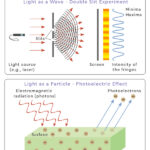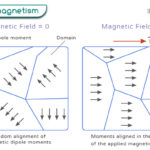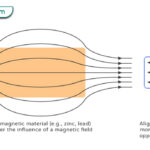Home / Physics / Electromagnetic Force

# Electromagnetic Force

Electromagnetism is a branch of physics that establishes the relationship between electricity and magnetism. A magnetic field affects moving charges, resulting in induced current. Moving charges produce magnetic fields. Therefore, the perception that magnetism and electricity are closely intertwined is correct.

## What is Electromagnetic Force

The electromagnetic force is the force of interaction between electrically charged particles, like electrons and protons, either stationary or moving. It consists of two distinct forces – electric force and magnetic force. It is one of the four fundamental forces of nature, including gravitational force, strong nuclear force, and weak nuclear force. Like the other fundamental forces, it has an infinite range. The electromagnetic force has a strength of 1/137 relative to the strong nuclear force. However, it is 1036 times stronger than the gravitational force.

How does Electromagnetic Force Work?

The electric force originates from the interaction between charged particles, whether they are stationary or moving. Oppositely charges attract each other while like charges repel. Charged particles give rise to an electric field. However, when the particles start to move, they generate a magnetic field which gives rise to a magnetic force. The electromagnetic force is manifested when the electric and magnetic fields interact with the charged particles. It is an exchange force, and the particles that carry the force are known as photons.

Who Discovered Electromagnetic Force?

In 1820, Danish physicist Hans Christian Oersted was the first to observe that the needle of a magnetic compass deflects when placed near a current-carrying wire. Later that year, he published his results in a pamphlet.

## Electromagnetic Force Examples

An effect of the electromagnetic force is that it is responsible for most interactions in nature. Here are some of its examples.

• Electrons moving around a nucleus
• The molecules of water
• Electromagnetic waves (E.g., light, radio wave, X-rays, and UV-rays)
• A current passing through a wire

## Electromagnetic Force Equation

### How to Calculate Electromagnetic Force

The electromagnetic force is a combination of the electric and the magnetic force. Hence, the equation is given by Lorentz force, whose formula is

$\vec{F} = q\vec{E} + q(\vec{v} \times \vec{B})$

Where,

$q \text{: Charge of the particle}\\ \vec{v} \text{: Velocity of the particle}\\ \vec{E} \text{: Electric field}\\ \vec{B} \text{: Magnetic field}$

## Applications of Electromagnetic Force

The electromagnetic force is applied in electromagnets, which find their way into many devices.

• Doorbells
• Hard-drives
• Speakers
• Microphones
• Home security system
• Generators
• Solenoid and toroid

## FAQs

Q.1. What is the difference between an electromagnet and a permanent magnet?

Ans. An electromagnet is a temporary magnet that can be magnetized by passing an electric current. A permanent magnet is one whose magnetism is permanent.

Q.2. What is the difference between electrostatic and electromagnetic force?

Ans. The difference between electrostatic and electromagnetic forces is that the former refers to the force between charges which are not moving relative to each other. On the other hand, electromagnetic force refers to electrostatic forces and other forces between moving charges and magnetic fields.

Q.3. How are electromagnetic forces used to generate electric power?

Ans. An electric generator is used to convert kinetic energy into electrical energy through electromagnetic induction. In this process, a turning shaft rotates a coil between two pole pieces of a magnet that generates an electric current.

Article was last reviewed on Friday, February 3, 2023

### Related articlesWave-Particle DualityPhotoelectric EffectFerromagnetismDiamagnetism